It looks like you're using Internet Explorer 11 or older. This website works best with modern browsers such as the latest versions of Chrome, Firefox, Safari, and Edge. If you continue with this browser, you may see unexpected results.

# Chemistry

Percent Composition

The percent composition of an element in a compound is calculated using the formula.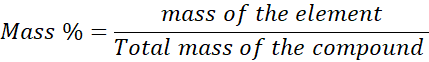Example 1

What is the percent composition of FeSO4?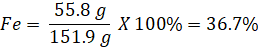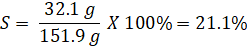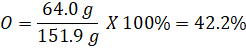The total percent composition adds up to 100% (36.7% + 21.1 % + 42.2% = 100%)

Determination of Empirical Formulas

The empirical formula is used to show the relative ratios of various atoms of a compound.

The masses of each element in a compound can be measured using experiments with that the empirical formula is derived as shown in the example below.

Find the empirical formula of a compound that contains 38.7% C, 16.2% H, and 45.1% N.

Step 1:

Assume 100 g of the compound then the number of moles of each element can be calculated as follows.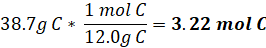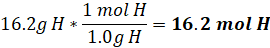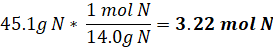Step 2: Determining mole ratio

C3.22H16.2N3.22

Step 3 : Divide all the moles by the smallest one found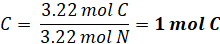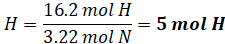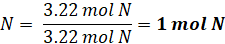Step 4: The empirical formula now can be written as follows

C1H5N1 = CH5N

Derivation of Molecular Formulas

Ethylene glycol has 38.7% C, 9.75% H, and Oxygen. Its molecular weight is 62.07g. Find the molecular formula of ethylene glycol.

Step 1

The amount of oxygen in the compound can be found using the following method since the total percent composition is equal to 100%

O = 100% - (38.7% + 9.75%) = 51.55%

Step 2

Assume 100 g of the compound then the number of moles of each element can be calculated as follows.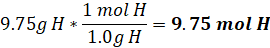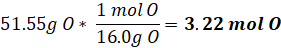Step 3

Divide all the moles by the smallest one found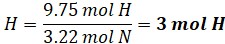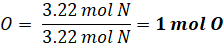Then the empirical formula becomes CH3O

Step 4

Calculating the ratio of molecular weight to empirical forumal weight

Molecular weight = 62.07g

Empirical formula weight = (1*12.0g)+(3*1.0g)+(1*16.0g) = 31.0g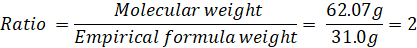Step 5

Multiply the mols of empirical formula by the ratio calculated in step 4

Then the molecular formula becomes C2H6O2